#### Howdy, Stranger!

We are about to switch to a new forum software. Until then we have removed the registration on this forum.

# 3D Dice Simulator

Wondering if anyone would be interested in a 3D Dice Simulator library. Please comment if you're interested. If there is sufficient support, I'll make a library - I have the basic framework ready. Thanks!

Tagged:

• None interested?

• Could you like provide a screen shot?

Could be interesting when someone makes a game with dices like settlers of catan

two six-sided dice are rolled to determine which hexes produce resource

https://en.m.wikipedia.org/wiki/Catan

• @Chrisir Thanks for your interest. Unfortunately, I do not, as yet, have a screenshot of it, but I will upload the (basic) code and a screenshot within a few days.

• Interesting. Is the idea a physics simulation of dice rolling as tumbling cubes falling onto a surface? If it is physics-oriented you thinking of using something like bRigid / jBullet, or just a simple gravity bounce algorithm and some basic collision detection?

• ``````    import peasy.*;
import peasy.org.apache.commons.math.*;
import peasy.org.apache.commons.math.geometry.*;

// PeasyCam cam;

Hexagon [] hexagons = new Hexagon ;

// resources (brick, lumber, wool, grain, and ore), represented by resource cards;

// land type
final int desert   = 0; // -> none   | decoration: palm tree
final int forest   = 1; // -> Wood   | decoration: tree, cottage
final int pasture  = 2; // -> Wool   | decoration: sheep
final int mountain = 3; // -> Ore
final int hills    = 4; // -> bricks
final int fields   = 5; // -> grain  | decoration: hald full during harvest

// this list reflects how many land types cards there are of every land type
int[] landTypesList = {
desert,
forest, forest, forest, forest,
pasture, pasture, pasture, pasture,
mountain, mountain, mountain,
hills, hills, hills,
fields, fields, fields, fields
};

// PImage textureForest;

// grass
final int numberOfStraws = 155;
PVector [] straws = new PVector[numberOfStraws];

// -------------------------------------------------------------

void setup() {
size(1400, 960, P3D);
background(#3064FA);

//cam = new PeasyCam (this, 100);
//cam.setMinimumDistance(50);
//cam.setMaximumDistance(500);

landTypesList=shuffleArray(landTypesList);

initHexagons();
initGrass();

//  textureForest = loadImage("t1.jpg"); //   ("forest1.png");
//
} // func

void draw() {
background(#3064FA);

camera(0, 533, 1410,
0, 811, 0,
0, 1, 0);

// test sphere at 0,0,0
//noStroke();
//fill(255, 2, 2);
//pushMatrix();
//translate(0, 0, 0);
//sphere(44);
//popMatrix();

// show the hexons
// translate (190, 90);
rotateY(map(mouseX, 0, width, 0, 2*TWO_PI));
rotateX(map(mouseY, 0, height, 0, 2*TWO_PI));
for (int i = 0; i < hexagons.length; i++) {
hexagons[i].display() ;
} // for
} // func

// ------------------------------------------

int[] shuffleArray (int[] arr) {

// printArray(arr);

int idx = arr.length;
while (idx > 1) {
int rnd = int ( random(idx) ) ;

int tmp = arr[--idx];
arr[idx] = arr[rnd];
arr[rnd] = tmp;
}
return arr;
}

void initHexagons() {
int i = 0;
//
int x = 0;
int y = -310;
for ( i = 0; i < 3; i++) {
hexagons[i] = new Hexagon ((x+1)*182+90+110, y, landTypesList[i]); //
x++;
} // for
//
x=0;
for ( i = 3; i < 7; i++) {
hexagons[i] = new Hexagon ((x+1)*182+0+110, y, landTypesList[i]);
x++;
} // for
x=0;
for ( i = 7; i < 12; i++) {
hexagons[i] = new Hexagon ((x)*182+90+110, y, landTypesList[i]); //
x++;
} // for
x=0;
for ( i = 12; i < 12+4; i++) {
hexagons[i] = new Hexagon ((x)*182+180+110, y, landTypesList[i]);
x++;
} // for
x=0;
for ( i = 12+4; i < 19; i++) {
hexagons[i] = new Hexagon ((x)*182+270+110, y, landTypesList[i]);
x++;
} // for
// --------------------------------------
for (i = 0; i < hexagons.length; i++) {
//  hexagons[i].y -= 0;
}
}// func

// ==========================================

class Hexagon {

float x, y, z;
color strokeColor ; //
PVector[] h1 = new PVector;
float angle=0.0;
int landType;

ArrayList<Tree> trees = new ArrayList();

// constructor
Hexagon( float xtemp, float ytemp,
int landTypetemp ) {
// constr

x=xtemp;
y=height;
z=ytemp;

landType=landTypetemp;
makeForest();

//strokeColor = strokecolortemp;

// for rotation
//  angle=angletemp;
// define the array
for (int i = 0; i < 6; i++)
h1[i] = new PVector();
//Set the points based on theta with a radius of 100
for (int i = 0; i < 6; i++) {
theta += TWO_PI/6.0;
h1[i].y =0;
}
}  // constr

void display() {

pushMatrix();
translate(x, y, z);
setColor();
stroke(111);

// Draw the hexagon using the points
beginShape();
//  setTexture();
for (int i = 0; i < 6; i++) {
vertex(h1[i].x, h1[i].y, h1[i].z);
}
endShape();

setDecoration();

popMatrix();
//
} // method

void setTexture() {
switch(landType) {

case desert:
break;

case forest:
// texture(textureForest);
break;

case pasture:
break;

case mountain:
break;

case hills:
break;

case fields:
break;

default:
println ("error 203: unknown land type: "+landType);
exit();
fill(0);
break;
} // switch
}// method

void setDecoration() {

switch(landType) {

case desert:

// sun
fill(#FFFF64);
noStroke();

pushMatrix();
translate(-50, -32, -40);
sphere(12);
popMatrix();

// sun rays

stroke(#FFFF64);
float r1=22;
float r2=40;

for (int i = -40; i < 160; i+=20) {

line(x1, -32, y1,
x2, -32+11, y2);
}

break;

case forest:
for (Tree t : trees)
t.display();
break;

case pasture:
// fill(#74FA84);
pushMatrix();
grass ();
translate (-33, 0, -33);
grass ();
translate (-22, 0, 55);
grass ();
popMatrix();
break;

case mountain:
//  fill(#869588);
break;

case hills:
// fill(#C18412);
break;

case fields:
fill(#D8C918);
break;

default:
println ("Unknown land type: "+landType);
exit();
fill(0);
break;
} // switch
}// method

void setColor() {

switch(landType) {

case desert:
fill(#FAE630);
break;

case forest:
fill(#1EC933);
break;

case pasture:
fill(#74FA84);
break;

case mountain:
fill(#869588);
break;

case hills:
fill(#C18412);
break;

case fields:
fill(#D8C918);
break;

default:
println ("unknown land type: "+landType);
exit();
fill(0);
break;
} // switch
}// method

void makeForest() {

color[] colorForest={
color(#31A010),
color(#289B06),
color(#2B8B0E),
color(#2B8B0D)};

for (int i = 0; i < 60; i++) {

float x1=random(-66, 66);
float y1=random(-42, -14);
float z1=random(-66, 66);
color colorTree = colorForest[int(random(colorForest.length))];

colorTree,
random(7, 18)));
}
}//method
//
} // class
//
// ===================================================================
// the Grass

void initGrass() {
for (int i = 0; i < numberOfStraws; i = i+1) {
straws[i] = new PVector ( random (4, 14), random (4, 14) );
}
}

void grass () {
stroke(0, 253, 2);

for (int i = 0; i < numberOfStraws; i = i+1) {
GrassOne(straws[i].x, straws[i].y);
}
GrassOne( 21, 21 );
GrassOne( 11, 33 );
GrassOne( 21, -22 );
GrassOne( -21, 21 );
GrassOne( -11, 33 );
GrassOne( -21, -22 );
GrassOne( 21, -21 );
GrassOne( 11, -33 );
GrassOne( 21, 22 );
GrassOne( -18, - 24 );
GrassOne( -17, -30 );
GrassOne( -19, -12 );
GrassOne( -8, - 4 );
GrassOne( -7, -3 );
GrassOne( -9, -7 );
}

void GrassOne (float X1, float Y1) {
//pushMatrix();
//translate();
X1=abs(X1);
Y1=abs(Y1);
stroke(0, 253, 2);
line (X1, 0, Y1,
X1, -9, Y1);
//popMatrix();
}

// =============================================================

class Tree {

float x, y, z, size;
color c;

Tree ( float x1, float y1, float z1,
color c1,
float size1) {

x=x1;
y=y1;
z=z1;

c=c1;
size=size1;
}//constr

void display() {
noStroke();
fill(c);

pushMatrix();
translate(x, y, z);

stroke(#C68214);
line(0, 0, 0,
0, -y, 0);

noStroke();
sphere(size);

popMatrix();
}
}
//
``````
• example of a small Settler of Catan Game

mouse to rotate

no game play....

• Cool.
@jeremydouglass I am actually using bRigid in the sketch. Unfortunately, my PC (laptop) has gotten infected with a virus and I'm unable to test anything right now. It may take two days before I can repair it (I'm no expert).

•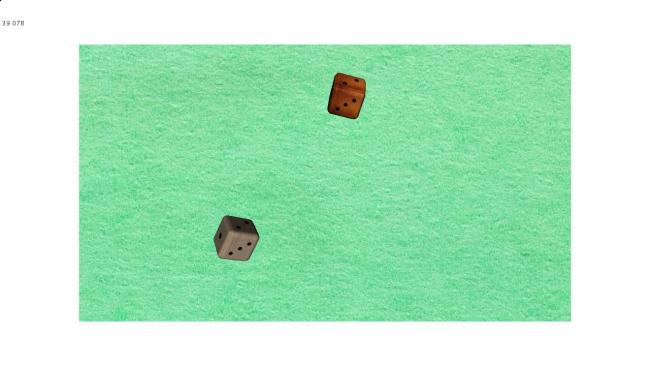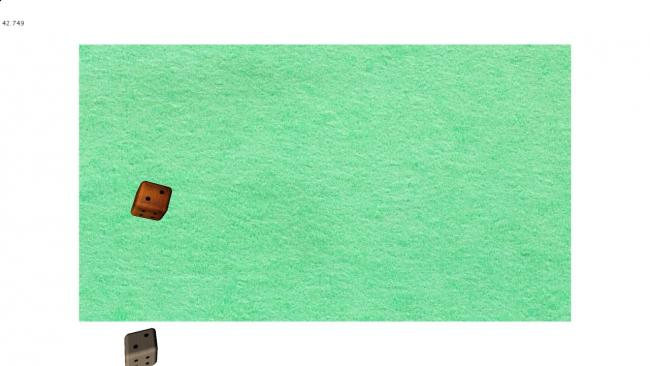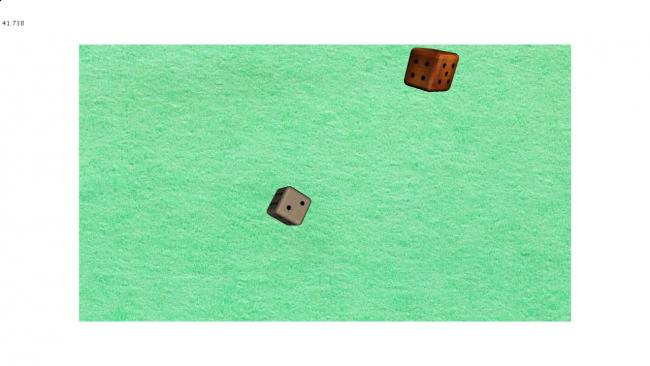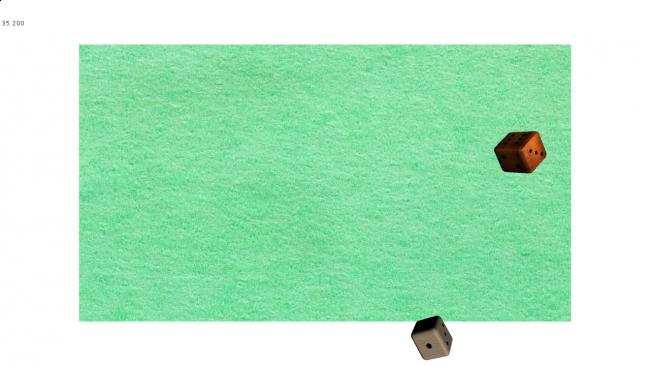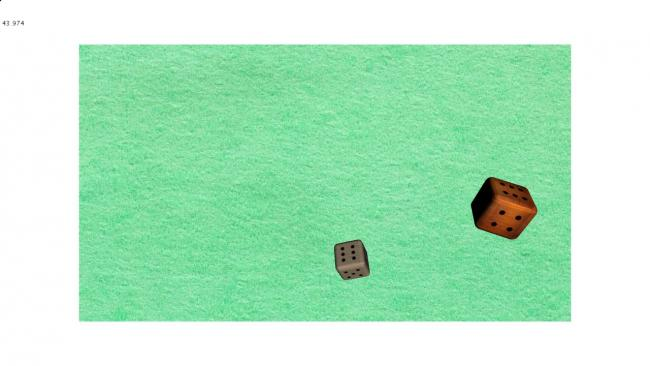Just some screenshots of the basic sketch.